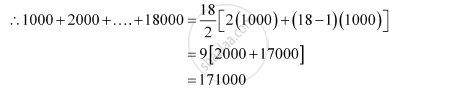CBSE (Arts) Class 11CBSE
Share
Notifications

View all notifications
Books Shortlist
Your shortlist is empty

# Shamshad Ali Buys a Scooter for Rs 22000. He Pays Rs 4000 Cash and Agrees to Pay the Balance in Annual Installment of Rs 1000 Plus 10% Interest on the Unpaid Amount. How Much Will the Scooter Cost Him? - CBSE (Arts) Class 11 - Mathematics

Login
Create free account

Forgot password?
ConceptArithmetic Progression (A.P.)

#### Question

Shamshad Ali buys a scooter for Rs 22000. He pays Rs 4000 cash and agrees to pay the balance in annual installment of Rs 1000 plus 10% interest on the unpaid amount. How much will the scooter cost him?

#### Solution

It is given that Shamshad Ali buys a scooter for Rs 22000 and pays Rs 4000 in cash.

∴Unpaid amount = Rs 22000 – Rs 4000 = Rs 18000

According to the given condition, the interest paid annually is

10% of 18000, 10% of 17000, 10% of 16000 … 10% of 1000

Thus, total interest to be paid = 10% of 18000 + 10% of 17000 + 10% of 16000 + … + 10% of 1000

= 10% of (18000 + 17000 + 16000 + … + 1000)

= 10% of (1000 + 2000 + 3000 + … + 18000)

Here, 1000, 2000, 3000 … 18000 forms an A.P. with first term and common difference both equal to 1000.

Let the number of terms be n.

∴ 18000 = 1000 + (n – 1) (1000)

⇒ n = 18∴ Total interest paid = 10% of (18000 + 17000 + 16000 + … + 1000)

= 10% of Rs 171000 = Rs 17100

∴Cost of scooter = Rs 22000 + Rs 17100 = Rs 39100

Is there an error in this question or solution?

#### APPEARS IN

NCERT Solution for Mathematics Textbook for Class 11 (2018 to Current)
Chapter 9: Sequences and Series
Q: 28 | Page no. 200
Solution Shamshad Ali Buys a Scooter for Rs 22000. He Pays Rs 4000 Cash and Agrees to Pay the Balance in Annual Installment of Rs 1000 Plus 10% Interest on the Unpaid Amount. How Much Will the Scooter Cost Him? Concept: Arithmetic Progression (A.P.).
S